A RANDOM GRAPH

The construction. A random (undirected) graph with n vertices is constructed in the following way: pairs of vertices are randomly selected one at a time in such a way that each pair has the same probability of being selected on any given occasion, and, each selection is made independently of previous selections. If the vertex pair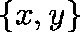is selected, then an edge is constructed which connects x and y.

Are multiple edges possible? Yes. For example, if the vertex pairwere to be selected subsequently, there would now be more than one edge connecting x and y: a multiple edge or cycle of length 2.

ASYMPTOTIC BEHAVIOUR

Suppose that m edges have been selected. We shall be concerned with the behaviour of the graph in the limit as n and m become large, but in such a way that.

The problem. Our problem is to determine the limiting probability that the graph is acyclic.

Motivation. Havas and Majewskipresent an algorithm for minimal perfect hashing

(used for memory-efficient storage and fast retrieval of items from static sets) based on this random graph. Their algorithm is optimal when the graph is acyclic.

WHY ACYCLIC?

Consider a set W of m words (or keys). Every bijection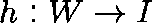, where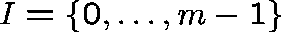, is called a minimal perfect hash function.

HM find hash functions of the formmap keys to integers (they identify the pair of vertices of the graph corresponding to the edge w) and g maps integers to I.

Given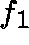and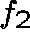, can g be chosen so that h is a bijection?

If the graph is acyclic then, yes, it is easy to construct g from h. Traverse the graph: if vertex w is reached from vertex u then setwhere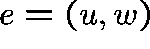.

EFFICIENCY

HM's algorithm generatesandat random until an acyclic graph is found: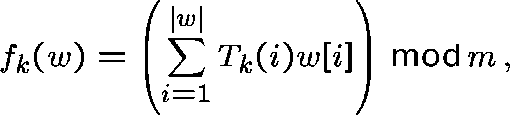where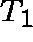andare tables of random integers and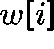denotes the i-th character (an integer) of key i.

The efficiency of the algorithm is determined by the probability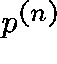that the graph is acyclic: the expected number of iterations needed to find an acyclic graph will be(typically between 2 and 3).

EVALUATINGConjecture. If n and m tend to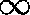in such a way that n=cm, where c is a positive constant, the limiting probability, p, that the graph is acyclic is given by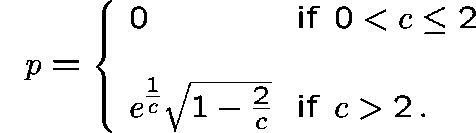``Proof''. Let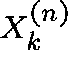be the number of cycles of length k and let. Following [HM] write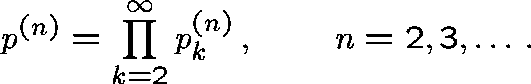Now let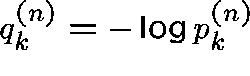, so thatand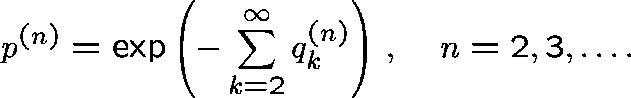Erdös and Renyishow that the distribution ofis asymptotically Poisson: in particular,whereIt follows thatSo, formally,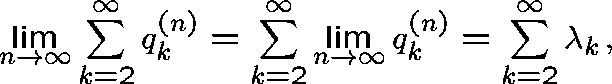and hencewhereBy Fatou's Lemma, we always havefrom which it follows immediately thatthis argument is valid even if the sum in (1) is divergent. Thus, we may deduce immediately that if,exists and equals 0.

The interesting case c>2 is also easily dealt with. When c>2, we have that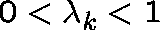and thatFrom Markov's inequality we haveand so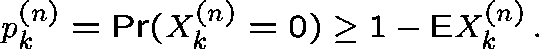But, by Lemma 2 of [HM], we know have that, for each fixed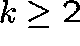,, as. In particular, for each fixed, the sequenceis bounded above by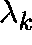. It follows that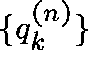is bounded above by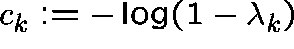. Further, since, we have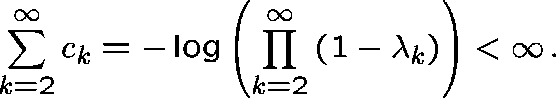Thus, by the Lebesgue Dominated Convergence Theorem, we have that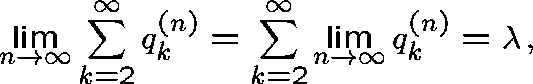and, hence, thatexists and is equal to.